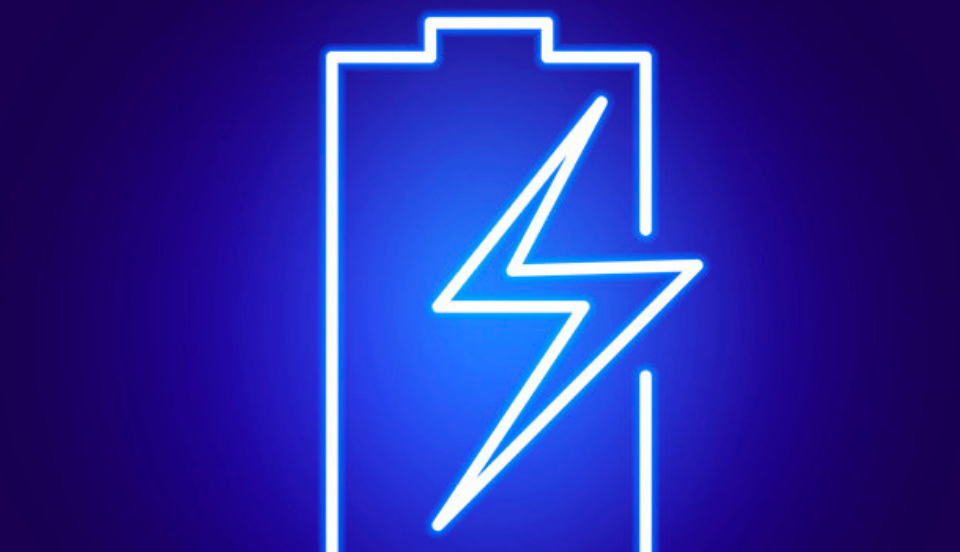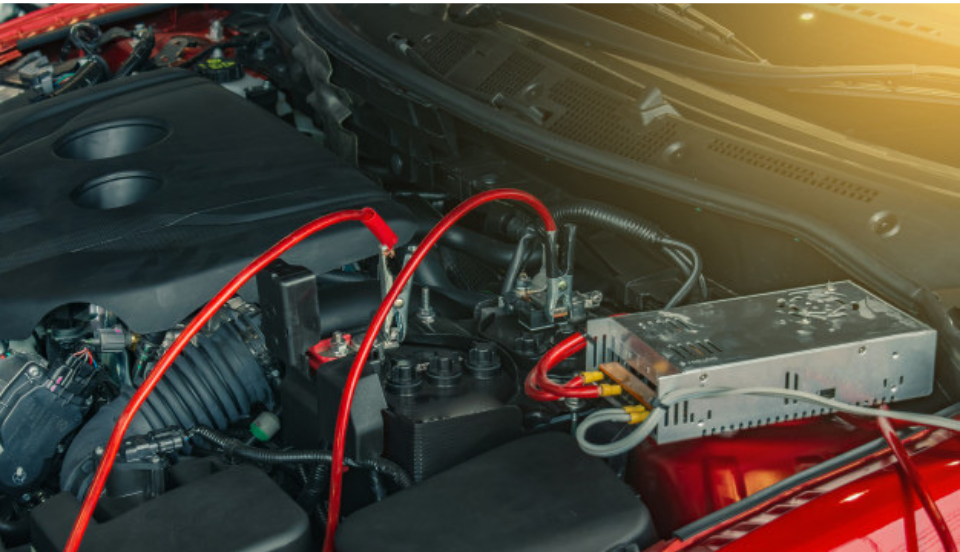# Differences Between an AMP and a JOULE

In the field of electronics, an amp and a joule are two diverse measuring units. For instance, if you are working on a project that requires you to build an electronic circuit then the first and the foremost thing that you need to be properly aware of is the difference between the various units of measurements. Two such units used for measurement in electronic equipment are amps and joules.

Has the search for an accurate explanation of the differences between an amp and a joule brought you here to our website? If yes, then your search comes to an end at this moment. In this post, you would come across a brief description of the differences between these two measuring units.

Amp:

An amp which is as sometimes also referred to as an ampere is a measuring unit used to measure the power of an electric current. For instance, if you go to buy a 24-volt battery jump starter what you would actually be concerned about is what would be the peak power that this particular jump started would be able to provide. It is this peak current that a battery provides that is measured in the form of peak amps.JOULE:

If we define a joule in simple terms then it would be a measuring unit of energy or work. It measures the force that is required to move any given thing over a particular distance. Joule is related to the work that would be required to move an ampere that would further help a car battery to start. When we calculate on the basis of per second then one joule would be equal to one watt of power given out i.e., one watt is the production of one joule for every second.

While joules could be converted to watts, amps cannot be converted to joules as they two vary in many respects as the units of measurement.

An amp measures the power, a joule is used to measure the total work done.

The symbol of an Amp is ‘A’ and the symbol of a joule is ‘J’. Keep these symbols in mind as they would turn out to be of use next time you are looking forward to knowing the value of amps and joules that a lithium jump starter has got to offer for your car.The relation that ampere shares with a coulomb are similar to the relation between a joule and a watt. For example, if one coulomb flows through your battery charger in a period of one second, then the current that is passing through your charging system would equal to one amp.

So, these were the differences between an amp and a joule. We have tried our best to make the differences between these two units of measurement extremely clear for you. With such a detailed post being made available to you by us, it would not at all be difficult for you to know about the total number of amps and joules that a particular car 12V car battery charger provides before you actually buy the same.

You have successfully subscribed!
20%off